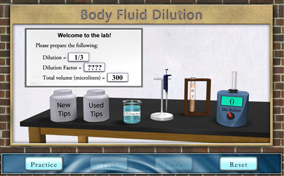# Body Fluid DilutionQuality patient care is a goal of all medical laboratory scientists. Accurate and precise methods and procedures are required to achieve the goal in laboratory testing. Most of the methods are characterized by an analytic measurement range (AMR) for all analytes (e.g., carbohydrates, glucose, hormones, lipids, electrolytes, etc.). Often the body fluid (BF) analyte concentration may exceed the range. For example, the glucose method AMR or range of linearity is 0 – 450 mg/dl, while an unknown glucose of 500 mg/dl would be above the range. In this situation, a dilution is needed to obtain the correct glucose results. Your challenge is to make various dilutions that meet the stated specifications.

### What is a body fluid dilution?

Generally, dilutions are weaker solutions which are made from stronger solutions. In the case with body fluids (serum, urine, blood, etc.), the concentration of the solutes or analytes in the BF will be decreased with the addition of the diluents, which may be water, saline or other specified diluents.

### What are the two components of a dilution?

A dilution consists of (1) a volume of the body fluid to be diluted and (2) a volume of the diluent to perform the dilution mixed together.

### What is the purpose of a body fluid dilution?

Body fluid dilutions are needed for accurate measurement of analytes in the body fluid. For example, in the chemistry laboratory, the analyte concentration in the BF may be greater than the method's AMR (range of linearity). In immunoserology, dilution is needed to determine the titer of antibodies in the BF, such as HepB antibody.

### What are the types of dilutions of body fluids?

A dilution may be a single or a multiple series of dilutions of the BF.

### What is the formula for a body fluid dilution?

The formula for determining the dilution of the BF in the total volume is: Dilution =
volume of body fluidvolume of body fluid + volume of diluent
Example: What is the dilution of the BF in the diluent when you have the following: 100 ml of BF that are added to 100 ml of diluent? Dilution =
100 µl of BF100 µl of BF + 100 µl of Diluent
Dilution =
100 µl<200 µl
Dilution = ½

### What is the dilution factor?

The dilution factor is the inverse of the dilution. For example, a 1/3 dilution (with the inverse being 3/1), results in the dilution factor being 3X.

### Describe the nomenclature of dilutions.

The term "nomenclature" means to give a word or phrase to a given object, property, or event. Dilutions require specific terminology to describe different solutions. Three different nomenclatures can be used to describe a dilution. For instance a 1/3 dilution can be stated in the following three ways: 1. 1 to 3 dilution of BF in diluent 2. 1 in 3 dilution of BF in diluent 3. 1 in 3 dilution of BF with diluent